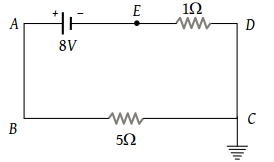# NEET Questions Solved

PMT - 2003

In the given circuit, the potential of the point E is(1) Zero

(2) –8 V

(3) –4/3 V

(4) 4/3 V

(3) The current in the circuit $=\frac{8}{5+1}=\frac{4}{3}$

Now ${V}_{C}-{V}_{E}=\frac{4}{3}×1$${V}_{E}=-\frac{4}{3}V$

Difficulty Level:

• 27%
• 27%
• 36%
• 12%